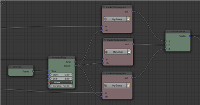## Animation Node experiment - cube grid

For this animation I created a 9x9x9 grid of cubes and animated the position of the cubes using the Animation-Nodes-Addon you can download the blend file here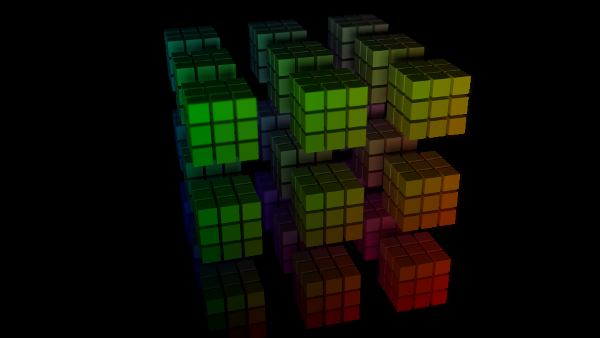When you download the blend file and look at the node setup it might look a bit confusing at the first glace but it's actually not so complicated when it's broken into sections.

first I generate 9x9x9 cubes using a object instance node and feed it to a loop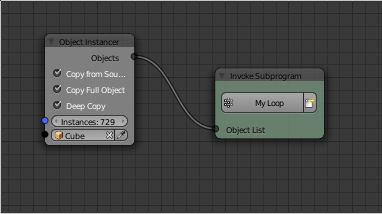in this loop I calculate the X, Y and Z coordinates of the single cubes. X coordinate is the index modulo 9, the Y coordinate is the index divided by 9, converted to an integer - which cuts away the parts after the comma - and the result modulo 9, the z coordinate is the index divided by 81 and then converted to an integer - again to get rid of the part after the comma.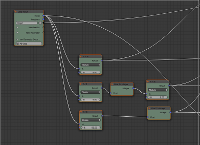The color of the cubes is calculated from the x, y and z coordinates by scaling them to the interval 0 to 1 and feeding them into an RGB-Color node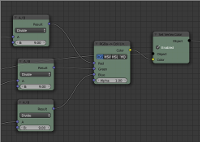to be able to offset 3x3x3 blocks of cubes I made a subgroup that divides a coordinate by 3, converts it to an integer and multiplies it with an ofset.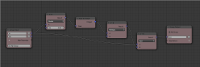this offsets are animated using an "Animate Float"-Node. The resulting x, y and z coordinates are then combined into a vector and then set to the object location useng a "Object Transofrm" node